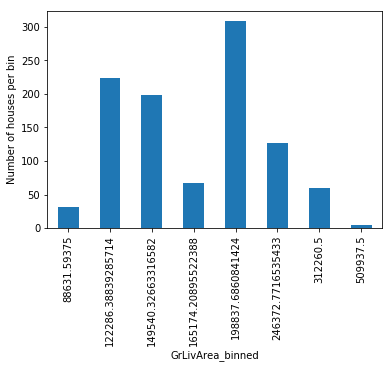# DecisionTreeDiscretiser#

The `DecisionTreeDiscretiser()` replaces numerical variables by discrete, i.e., finite variables, which values are the predictions of a decision tree. The method is based on the winning solution of the KDD 2009 competition:

In the original article, each feature in the challenge dataset was re-coded by training a decision tree of limited depth (2, 3 or 4) using that feature alone, and letting the tree predict the target. The probabilistic predictions of this decision tree were used as an additional feature, that was now linearly (or at least monotonically) correlated with the target.

According to the authors, the addition of these new features had a significant impact on the performance of linear models.

Example

In the following example, we re-code 2 numerical variables using decision trees.

First we load the data and separate it into train and test:

```import numpy as np
import pandas as pd
import matplotlib.pyplot as plt
from sklearn.model_selection import train_test_split

from feature_engine.discretisation import DecisionTreeDiscretiser

# Separate into train and test sets
X_train, X_test, y_train, y_test =  train_test_split(
data.drop(['Id', 'SalePrice'], axis=1),
data['SalePrice'], test_size=0.3, random_state=0)
```

Now we set up the discretiser. We will optimise the decision tree’s depth using 3 fold cross-validation.

```# set up the discretisation transformer
disc = DecisionTreeDiscretiser(cv=3,
scoring='neg_mean_squared_error',
variables=['LotArea', 'GrLivArea'],
regression=True)

# fit the transformer
disc.fit(X_train, y_train)
```

With `fit()` the transformer fits a decision tree per variable. Then, we can go ahead replace the variable values by the predictions of the trees:

```# transform the data
train_t= disc.transform(X_train)
test_t= disc.transform(X_test)
```

The `binner_dict_` stores the details of each decision tree.

```disc.binner_dict_
```
```{'LotArea': GridSearchCV(cv=3, error_score='raise-deprecating',
estimator=DecisionTreeRegressor(criterion='mse', max_depth=None,
max_features=None,
max_leaf_nodes=None,
min_impurity_decrease=0.0,
min_impurity_split=None,
min_samples_leaf=1,
min_samples_split=2,
min_weight_fraction_leaf=0.0,
presort=False, random_state=None,
splitter='best'),
iid='warn', n_jobs=None, param_grid={'max_depth': [1, 2, 3, 4]},
pre_dispatch='2*n_jobs', refit=True, return_train_score=False,
scoring='neg_mean_squared_error', verbose=0),
'GrLivArea': GridSearchCV(cv=3, error_score='raise-deprecating',
estimator=DecisionTreeRegressor(criterion='mse', max_depth=None,
max_features=None,
max_leaf_nodes=None,
min_impurity_decrease=0.0,
min_impurity_split=None,
min_samples_leaf=1,
min_samples_split=2,
min_weight_fraction_leaf=0.0,
presort=False, random_state=None,
splitter='best'),
iid='warn', n_jobs=None, param_grid={'max_depth': [1, 2, 3, 4]},
pre_dispatch='2*n_jobs', refit=True, return_train_score=False,
scoring='neg_mean_squared_error', verbose=0)}
```

With tree discretisation, each bin, that is, each prediction value, does not necessarily contain the same number of observations.

```# with tree discretisation, each bin does not necessarily contain
# the same number of observations.
train_t.groupby('GrLivArea')['GrLivArea'].count().plot.bar()
plt.ylabel('Number of houses')
```Note

Our implementation of the `DecisionTreeDiscretiser()` will replace the original values of the variable by the predictions of the trees. This is not strictly identical to what the winners of the KDD competition did. They added the predictions of the features as new variables, while keeping the original ones.

## More details#

Check also for more details on how to use this transformer:

All notebooks can be found in a dedicated repository.